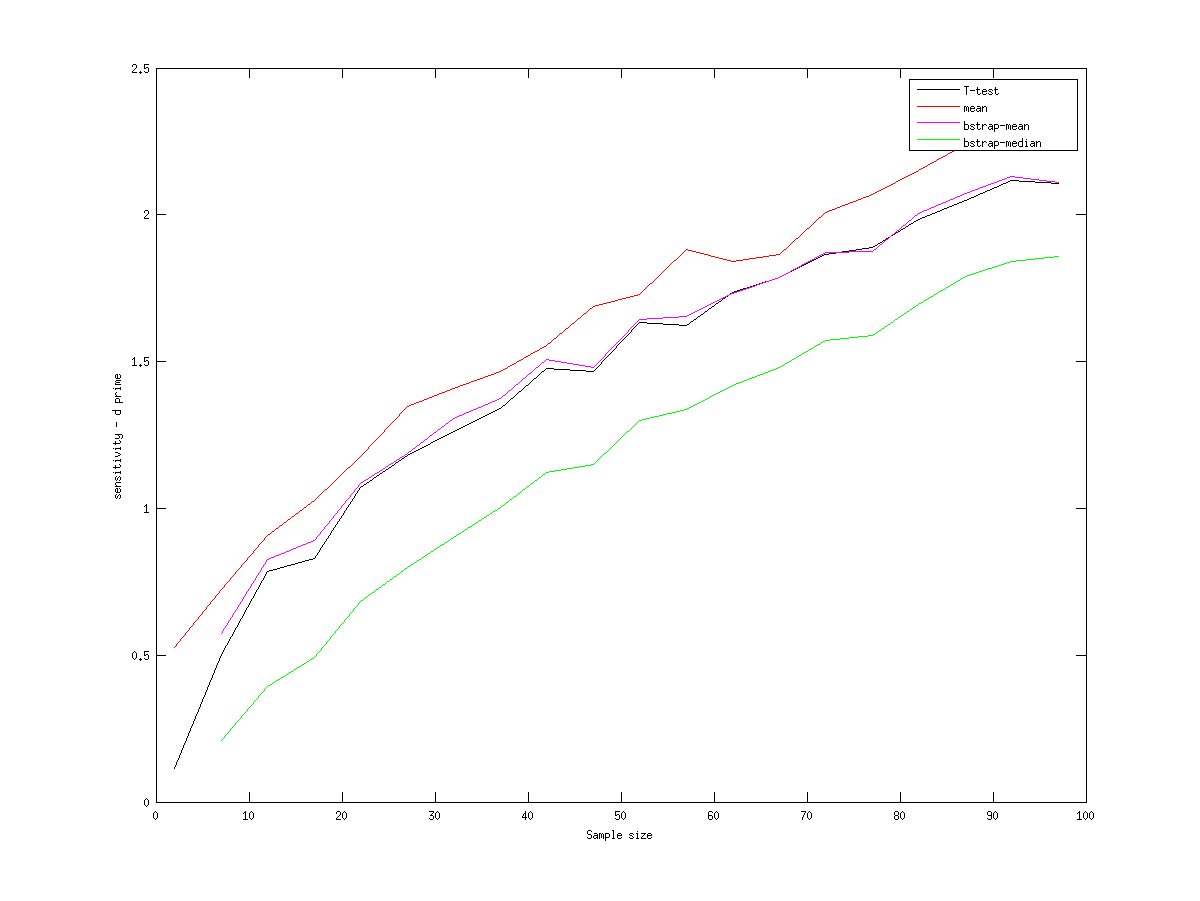So, previously on this blog (here, and here) I was playing around with the bootstrap as a way of testing if two samples are drawn from a different underlying distribution, by simulating samples with known differences and throwing different tests at the samples. The problem was that I was using the wrong bootstrap test. Tim was kind enough to look at what I’d done and point out that I should have concatenated my two sets of numbers and the pulled two samples from that set, calculated the mean difference and then used that statistic to constructed a probability distribution function against which I could compare my measured statistic (ie the difference of means) to perform a hypothesis test (viz. ‘what are the chances that I could have got this difference of means if the two distributions are not different?’). For people who prefer to think in code, the corrected bootstrap is at the end of this post.

Using the correct bootstrap method, this is what you get:So what you can see is that, basically, the bootstrap is little improvement over the t-test. Perhaps a marginal amount. As Cosma pointed out, the ex-gaussian / reaction time distributions I’m using look pretty normal at lower sample sizes, so it isn’t too surprising that the t-test is robust. Using the median rather than the mean damages the sensitivity of the bootstrap (contra my previous, erroneous, results). My intuition is that the mean, as a statistic, is influenced by the whole distribution in a way the median isn’t, so it a better summary statistic (statisticians, you can tell me if this makes sense). The mean test is far more sensitive, but, as discussed previously, this is because it has an unacceptably high false alarm rate which is insufficiently penalised by d-prime.

Update: Cosma’s notes on the bootstrap are here and recommened if you want the fundamentals and are already degree-level comfortable with statistical theory.

Corrected boostrap function:

```function H=bootstrap(s1,s2,samples,alpha,method)

difference=mean(s2)-mean(s1);

for i=1:samples

sstar=[s1 s2];

boot1=sstar(ceil(rand(1,length(s1))*length(sstar)));
boot2=sstar(ceil(rand(1,length(s2))*length(sstar)));

if method==1
a(i)=mean(boot1)-mean(boot2);
else
a(i)=median(boot1)-median(boot2);
end

end

CI=prctile(a,[100*alpha/2,100*(1-alpha/2)]);

H = CI(1)>difference | CI(2)